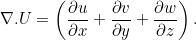# The Stokes equations for chyme flowing down the gut

Equip the gut with a coordinate system with coordinates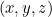and let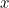be the direction pointing along the length of the gut. Write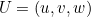for the velocity of the chyme. Here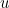is the component of the velocity in the direction of the-axis andand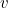are the velocity components in the other two directions. Write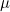for the viscosity of the chyme (a measure of its stickiness) and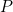for the pressure forcing the chyme to flow down the intestine. The Stokes equations relate velocity, pressure and viscosity as follows: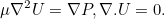Here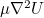is the vector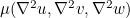, where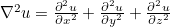(similarly for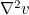and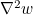).

The expression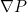stands for the vector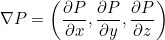and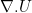is defined as